Processing ......FreeComputerBooks.com Links to Free Computer, Mathematics, Technical Books all over the World

Discrete Mathematics: An Open Introduction
Top Free Computer Networking Books 🌠 - 100% Free or Open Source!
• Title: Discrete Mathematics: An Open Introduction
• Author(s) Oscar Levin
• Publisher: CreateSpace (December 31, 2018); eBook (3rd Edition, 12/29/2019)
• Paperback: 407 pages
• eBook: HTML and PDF (412 pages)
• Language: English
• ASIN: 1792901690
• ISBN-13: 978-1792901690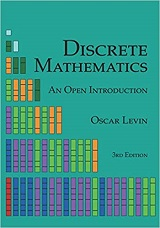Book Description

This third edition brings improved exposition, a new section on trees, and a bunch of new and improved exercises.

This gentle introduction to discrete mathematics is written for first and second year math majors, especially those who intend to teach. The text began as a set of lecture notes for the discrete mathematics course at the University of Northern Colorado.

This course serves both as an introduction to topics in discrete math and as the "introduction to proof" course for math majors. The course is usually taught with a large amount of student inquiry, and this text is written to help facilitate this.

Four main topics are covered: counting, sequences, logic, and graph theory. Along the way proofs are introduced, including proofs by contradiction, proofs by induction, and combinatorial proofs.

The book contains over 360 exercises, including 230 with solutions and 130 more involved problems suitable for homework. There are also Investigate! activities throughout the text to support active, inquiry based learning.

While there are many fine discrete math textbooks available, this text has the following advantages:

• It is written to be used in an inquiry rich course.
• It is written to be used in a course for future math teachers.
• It is open source, with low cost print editions and free electronic editions.
• Oscar Levin is an Associate Professor at the University of Northern Colorado in the School of Mathematical Sciences. He has taught mathematics at the college level for over 10 years and has received multiple teaching awards. He received his Ph.D. in mathematics from the University of Connecticut in 2009.
Reviews, Ratings, and Recommendations: Related Book Categories: Read and Download Links:Similar Books:
•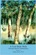A Cool Brisk Walk Through Discrete Mathematics (Stephen Davies)

This is a completely and forever free and open source educational materials dedicated to the mathematics that budding computer science practitioners actually need to know. They feature the fun and addictive teaching of award-winning lecturer!

•Discrete Structures for Computer Science: Counting, Recursion

This book provides a broad introduction to some of the most fascinating and beautiful areas of discrete mathematical structures with examples in logic, applications of the principle of inclusion and exclusion and finally the pigeonhole principal.

•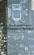Applied Discrete Structures, Fundamentals (Al Doerr, et al)

This book contains most of the fundamental concepts taught in a one semester course in discrete mathematics which is a required course for students in Computer Science, Mathematics and Information Technology.

•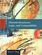Discrete Structures, Logic, and Computability (James L. Hein)

This book contains programming experiments that are designed to reinforce the learning of discrete mathematics, logic, and computability, provides introductions to those ideas and techniques to understand and practice the art and science of computing.

•A Spiral Workbook for Discrete Mathematics (Harris Kwong)

This is a book on discrete mathematics with an emphasis on motivation. It explains and clarifies the unwritten conventions in mathematics, and guides the students through a detailed discussion on how a proof is revised from its draft to a final polished form.

•Mathematics for Computer Science (Eric Lehman, et al)

This book covers elementary discrete mathematics for computer science and engineering. It emphasizes mathematical definitions and proofs as well as applicable methods. explores the topics of basic combinatorics, number and graph theory, logic and proof techniques.

•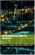Mathematics for Computer Scientists (Gareth J. Janacek, et al)

The aim of this book is to present some the basic mathematics that is needed by computer scientists. The reader is not expected to be a mathematician and we hope will find what follows useful.

•Lists, Decisions and Graphs - With an Introduction to Probability

In this book, four basic areas of discrete mathematics are presented: Counting and Listing (Unit CL), Functions (Unit Fn), Decision Trees and Recursion (Unit DT), and Basic Concepts in Graph Theory (Unit GT).

•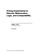Prolog Experiments in Discrete Math, Logic, and Computability

This book contains programming experiments that are designed to reinforce the learning of discrete mathematics, logic, and computability. The Prolog programming language is the tool used for the experiments in this book.

•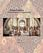Isomorphism - Mathematics of Programming (Xinyu Liu)

This book introduces the mathematics behind computer programming. It intents to tell: programming is isomorphic to mathematics. Just like in art and music, there are interesting stories and mathematicians behind the great minds.

•Discrete Mathematics for Computer Science (Jean Gallier)

This book gives an introduction to discrete mathematics for beginning undergraduates. One of original features of this book is that it begins with a presentation of the rules of logic as used in mathematics. Many examples of formal and informal proofs are given.

•Discrete Mathematics for Computer Science Students

This book gives thorough coverage to topics that have great importance to computer scientists and provides a motivating computer science example for each math topic. Contents: Counting; Cryptography and Number Theory; Reflections on Logic and Proof.

•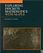Exploring Discrete Mathematics With Maple (Kenneth H. Rosen)

This is is a supplement in discrete mathematics to concentrate on the computational aspects of the computer algebra system Maple. Detailed instructions for the use of Maple are included in an introductory chapter and in each subsequent chapter.

Book Categories
 :All CategoriesTop Free BooksRecent BooksMiscellaneous BooksComputer EngineeringComputer LanguagesComputer ScienceData Science/DatabasesJava and Java EE (J2EE)Linux and UnixMathematicsMicrosoft and .NETMobile ComputingNetworking and CommunicationsSoftware EngineeringSpecial TopicsWeb Programming
Other Categories